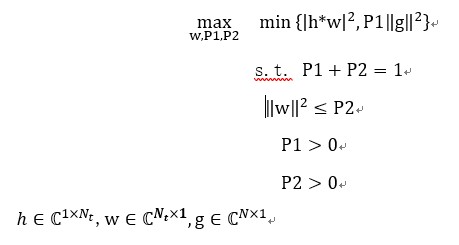# Invalid operation: min( {positive convex}, {real affine} )

I want to ask a question. In my opinion, the first term of Min function is a convex function and the second term is a linear function. Why can’t CVX be used to solve it directly?codes
Nt=4;
N=4;
h=(randn(1,Nt)+1irandn(1,Nt))/sqrt(2);
g=(randn(N,1)+1i
randn(N,1))/sqrt(2);
SNR_rd=(sum(abs(g)))^2;
cvx_begin
variables P1 P2 w(Nt,1);
maximize min((norm(hw))^2,P2SNR_rd)
subject to
power(norm(w),2)<=P1;
P1+P2==1;
P1>eps;
P2>eps;
cvx_end
Disciplined convex programming error:
Invalid operation: min( {positive convex}, {real affine} )

In order to “maximize” your objective function should be concave. The minimum of a convex function and a linear function is not concave. (It is not convex either, by the way).

Thank your very much. How to solve this problem?